Refer to our Texas Go Math Grade 5 Answer Key Pdf to score good marks in the exams. Test yourself by practicing the problems from Texas Go Math Grade 5 Module 13 Assessment Answer Key.

Concepts and Skills

Convert. TEKS 5.7.A

Question 1.
5mi = ___ yd
We know that,
1 mile = 1,760 yards
So,
5 miles = 5 × 1,760 yards
= 8,800 yards
Hence, from the above,
We can conclude that
5 miles = 8,800 yards

Question 2.
48qt = __ gal
We know that,
1 quart = $$\frac{1}{4}$$ gallons
So,
48 quarts = 48 × $$\frac{1}{16}$$ gallons
= 3 gallons
Hence, from the above,
We can conclude that
48 quarts = 3 gallons

Question 3.
9 T = __ lb
We know that,
1 Ton = 2,000 Pounds
So,
9 Tons = 9 × 2,000 Pounds
= 18,000 Pounds
Hence, from the above,
We can conclude that
9 Tons = 18,000 Pounds

Question 4.
5 kg = __ g
We know that,
1 kg = 1,000 grams
So,
5 kg = 5 × 1,000 grams
= 5,000 grams
Hence, from the above,
We can conclude that
5 kg = 5,000 grams

Question 5.
12 ft = __ yd
We know that,
1 feet = $$\frac{1}{3}$$ yard
So,
12 feet = 12 × $$\frac{1}{3}$$ yard
= 4 yards
Hence, from the above,
We can conclude that
12 feet = 4 yards

Question 6.
8,000 mm = __ m
We know that,
1 mim = $$\frac{1}{1,000}$$ m
So,
8,000 mm = 8,000 × $$\frac{1}{1,000}$$ m
= 8 m
Hence, from the above,
We can conclude that
8,000 mm = 8 m

Compare. Write <, >, or =. TEKS 5.7.A

Question 7.
96 fl oz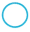13 c
The given units are: Fluid ounces, Cups
Now,
We know that,
1 fluid ounce = $$\frac{1}{8}$$ cups
So,
96 fluid ounces = 96 × $$\frac{1}{8}$$ cups
= 12 cups
So,
12 cups < 13 cups
Hence, from the above,
We can conclude thatQuestion 8.
4 L4,000 mL
The given units are: Liter, Milliliter
Now,
We know that,
1 L = 1,000 mL
So,
4 L = 4 × 1,000 mL
= 4,000 mL
So,
4,000 mL = 4,000 mL
Hence, from the above,
We can conclude thatQuestion 9.
8 yd288 in.
The given units are: Yard, Inch
Now,
We know that,
1 yard = 36 inches
So,
8 yard = 8 × 36 inches
= 288 inches
So,
288 inches = 288 inches
Hence, from the above,
We can conclude thatSolve. TEKS 5.7.A

Question 10.
A large freight elevator can carry a maximum of 9,800 pounds. Movers loaded equipment on the elevator that weighs 4 tons. How many more pounds of equipment can the elevator carry?
It is given that
A large freight elevator can carry a maximum of 9,800 pounds. Movers loaded equipment on the elevator that weighs 4 tons
Now,
We know that,
1 Ton = 2,000 Pounds
So,
4 Tons = 4 × 2,000
= 8,000 Pounds
So,
The number of more pounds of equipment the elevator can carry = 9,800 – 8,000
= 1,800 pounds
Hence, from the above,
We can conclude that
The number of more pounds of equipment the elevator can carry is: 1,800 pounds

Question 11.
A standard coffee mug has a capacity of 16 fluid ounces. If Annie needs to fill 26 mugs with coffee, how many total quarts of coffee does she need?
It is given that
A standard coffee mug has a capacity of 16 fluid ounces and Annie needs to fill 26 mugs with coffee
Now,
We know that,
1 cup = 8 fluid ounces
1 quart = 32 fluid ounces
So,
26 mugs = 26 × 8 fluid ounces
= 208 fluid ounces
So,
The total number of fluid ounces of coffee does Annie needs = 208 + 16
= 224 fluid ounces
The total number of quarts of coffee does Annie needs = 224 × $$\frac{1}{32}$$
= 7 quarts
Hence, from the above,
We can conclude that
The total number of quarts of coffee does Annie needs is: 7 quarts

Texas Test Prep

Question 12.
Howard cuts 80 centimeters off a 1.5-meter board. How much of the board does Howard have left? TEKS 5.7.A
(A) 230 centimeters
(B) 70 centimeters
(C) 20 centimeters
(D) 1,420 centimeters
It is given that
Howard cuts 80 centimeters off a 1.5-meter board
Now,
We know that,
1 m = 100 cm
So,
1.5 m = 1.5 × 100
= 150 cm
So,
The amount of the board does Howard have left = 150 – 80
= 70 cm
Hence, from the above,
We can conclude that
The amount of the board does Howard have left is: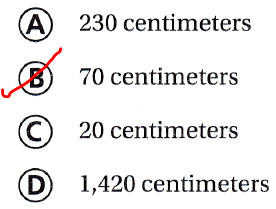Question 13.
Mr. Carter fills a tub with water so he can give his dog a bath. Which is the most appropriate unit for measuring the capacity of a tub? TEKS 5.7
(A) fluid ounce
(B) pound
(C) pint
(D) gallon
It is given that
Mr. Carter fills a tub with water so he can give his dog a bath
Hence, from the above,
We can conclude that
The most appropriate unit for measuring the capacity of a tub is:Question 14.
Milton purchases a 5-gallon aquarium for his bedroom. To fill the aquarium with water, he uses a container with a capacity of 1 quart. How many times will Milton fill and empty the container before the aquarium is full? TEKS 5.7.A
(A) 10
(B) 15
(C) 20
(D) 25
It is given that
Milton purchases a 5-gallon aquarium for his bedroom. To fill the aquarium with water, he uses a container with a capacity of 1 quart
Now,
We know that,
1 gallon = 4 quarts
So,
5 gallons = 5 × 4 quarts
= 20 quarts
So,
The number of times will Milton fill and empty the container before the aquarium is full is: 20 quarts
Hence, from the above,
We can conclude that
The number of times will Milton fill and empty the container before the aquarium is full is: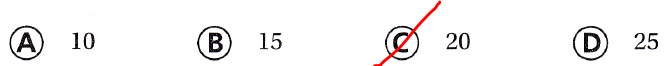Question 15.
Alfred uses the same tool to measure the distance across a sidewalk and the distance across town. Which tool does he use? TEKS 5.7
(A) odometer
(B) metric ruler
(C) medical scale
(D) trundle wheel
It is given that
Alfred uses the same tool to measure the distance across a sidewalk and the distance across town.
Hence, from the above,
We can conclude that
The tool that Alfred will use is: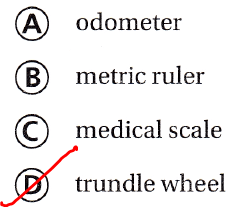Question 16.
A girl walks 5,000 meters in one hour. If the girl walks at the same speed for 4 hours, how many kilometers will she walk? TEKS 5.7.A
Record your answer and fill in the bubbles on the grid. Be sure to use the correct place value.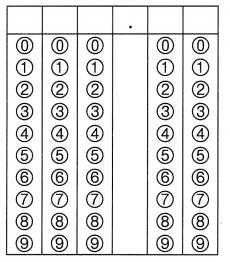It is given that
A girl walks 5,000 meters in one hour and the girl walks at the same speed for 4 hours
So,
The number of meters the girl walked = 5,000 × 4
= 20,000 meters
Now,
We know that,
1 m = $$\frac{1}{1,000}$$ kilometers
So,
The number of kilometers the girl walked = $$\frac{20,000}{1,000}$$
= 20 kilometers
Hence, from the above,
We can conclude that
The number of kilometers the girl walked is: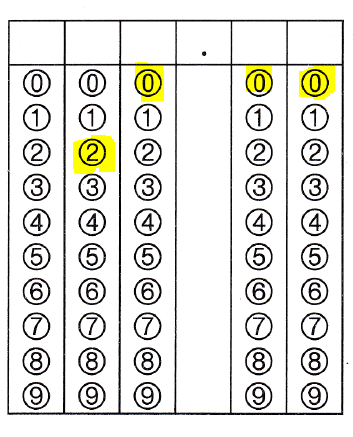Scroll to Top